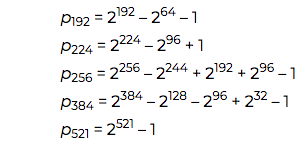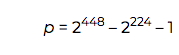# Tricks for Modular Arithmetic for NIST Primes

### Learn some tricks for modular arithmetic for NIST primes.

· AI Zone · Tutorial
Save
3.06K Views

The US National Institute of Standards and Technology (NIST) originally recommended 15 elliptic curves for use in elliptic curve cryptography . Ten of these are over a field of size 2. The other five are over prime fields. The sizes of these fields are known as the NIST primes.

The NIST curves over prime fields are named after the number of bits in the prime: the name is "P-" followed by the number of bits. The primes themselves are named p with a subscript for the number of bits.

The five NIST primes are:The largest of these, p521, is a Mersenne prime, and the rest are generalized Mersenne primes.

Except for p521, the exponents of 2 in the definitions of the NIST primes are all multiples of 32 or 64. This leads to efficient tricks for arithmetic modulo these primes carried out with 32-bit or 64-bit integers. You can find pseudocode implementations for these tricks in Mathematical routines for the NIST prime elliptic curves.

The elliptic curve Ed448 "Goldilocks " was not part of the original set of recommended curves from NIST but has been added. It employs a multiplication trick in the same spirit as the routines referenced above, but simpler. Ed448 useswhich has the special form φ² - φ - 1 where φ = 2 224. This enables a trick known as Karatsuba multiplication. More on that here.

## Related Posts

 FIPS PUB 186-4. This publication is dated 2013, but the curve definitions are older. I haven’t found for certain when the curves were defined. I’ve seen one source that says 1997 and another that says 1999.

Topics:
cryptography, number theory, ai, ai math, modular arithmetic, nist primes

Published at DZone with permission of John Cook, DZone MVB.

Opinions expressed by DZone contributors are their own.